Скачать презентацию Grade 6 — Claim 1 Target D Claim

7af3bcca6388b663c293feaee299d06b.ppt

• Количество слайдов: 33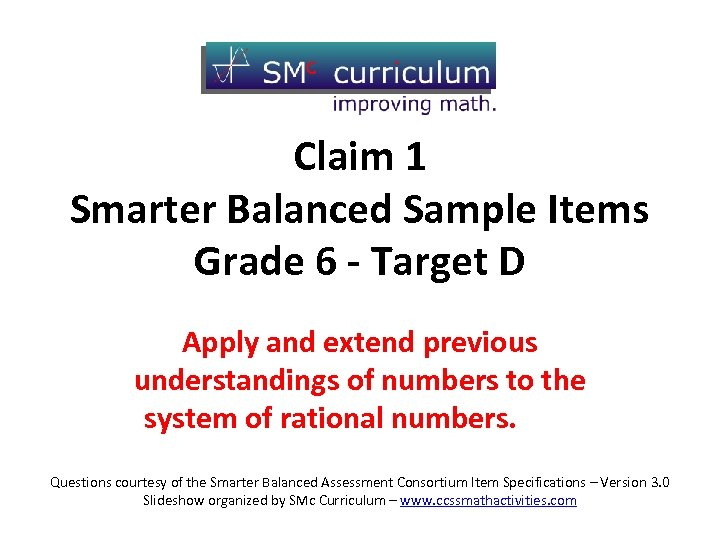Grade 6 - Claim 1 Target D Claim 1 Smarter Balanced Sample Items Grade 6 - Target D Apply and extend previous understandings of numbers to the system of rational numbers. Questions courtesy of the Smarter Balanced Assessment Consortium Item Specifications – Version 3. 0 Slideshow organized by SMc Curriculum – www. ccssmathactivities. com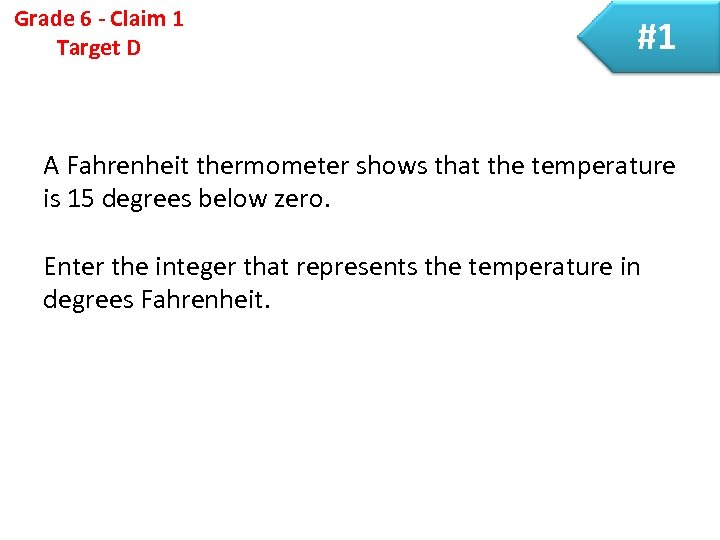Grade 6 - Claim 1 Target D #1 A Fahrenheit thermometer shows that the temperature is 15 degrees below zero. Enter the integer that represents the temperature in degrees Fahrenheit.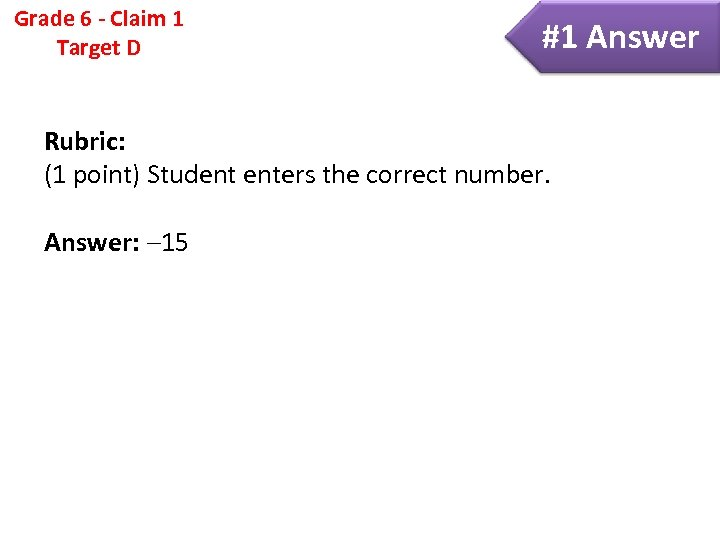Grade 6 - Claim 1 Target D #1 Answer Rubric: (1 point) Student enters the correct number. Answer: 15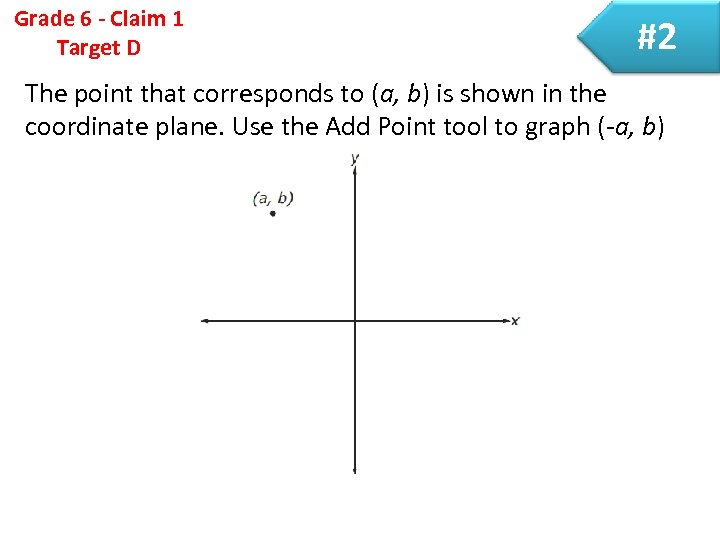Grade 6 - Claim 1 Target D #2 The point that corresponds to (a, b) is shown in the coordinate plane. Use the Add Point tool to graph (-a, b)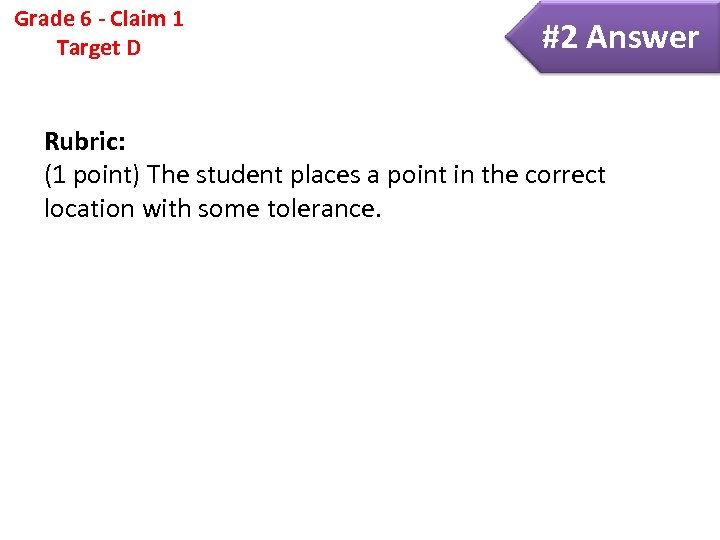Grade 6 - Claim 1 Target D #2 Answer Rubric: (1 point) The student places a point in the correct location with some tolerance.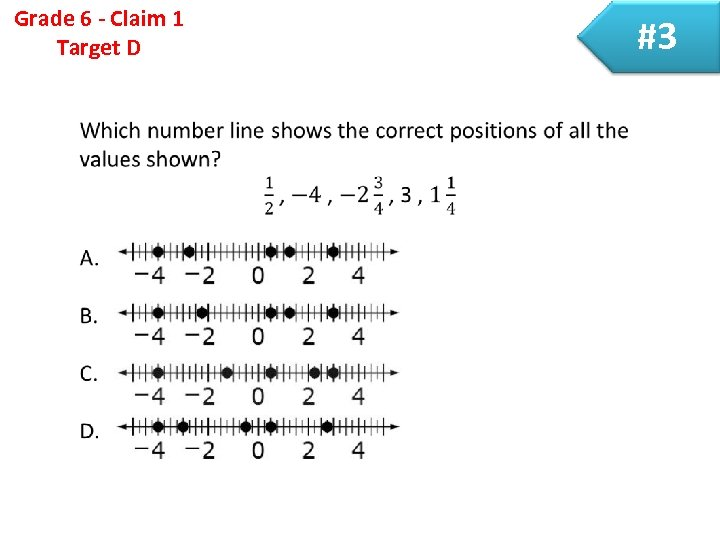Grade 6 - Claim 1 Target D #3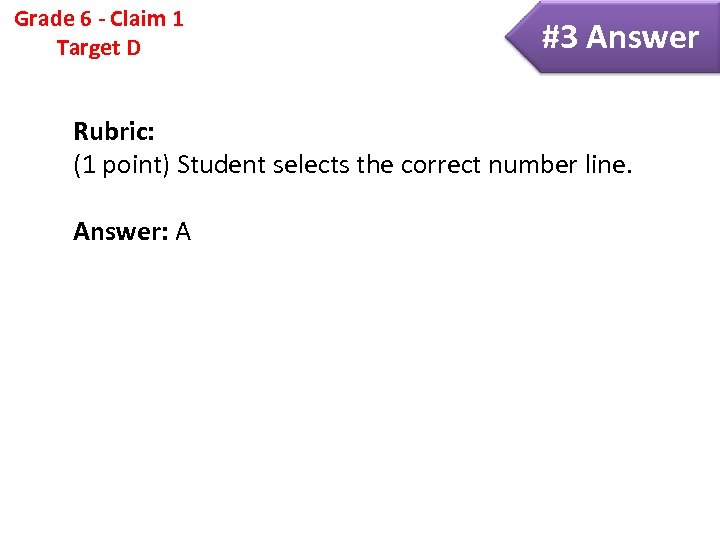Grade 6 - Claim 1 Target D #3 Answer Rubric: (1 point) Student selects the correct number line. Answer: A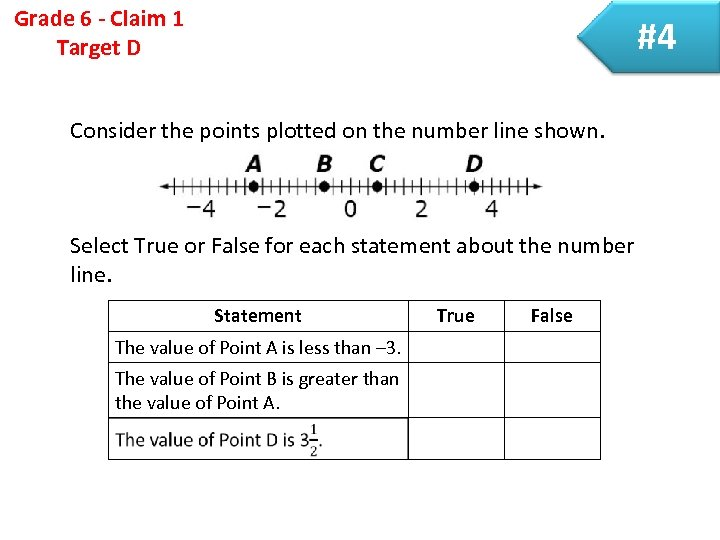Grade 6 - Claim 1 Target D #4 Consider the points plotted on the number line shown. Select True or False for each statement about the number line. Statement The value of Point A is less than – 3. The value of Point B is greater than the value of Point A. True False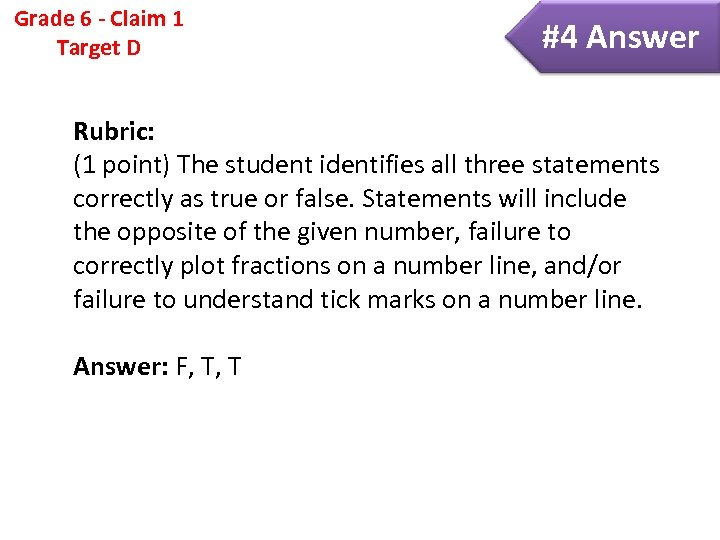Grade 6 - Claim 1 Target D #4 Answer Rubric: (1 point) The student identifies all three statements correctly as true or false. Statements will include the opposite of the given number, failure to correctly plot fractions on a number line, and/or failure to understand tick marks on a number line. Answer: F, T, T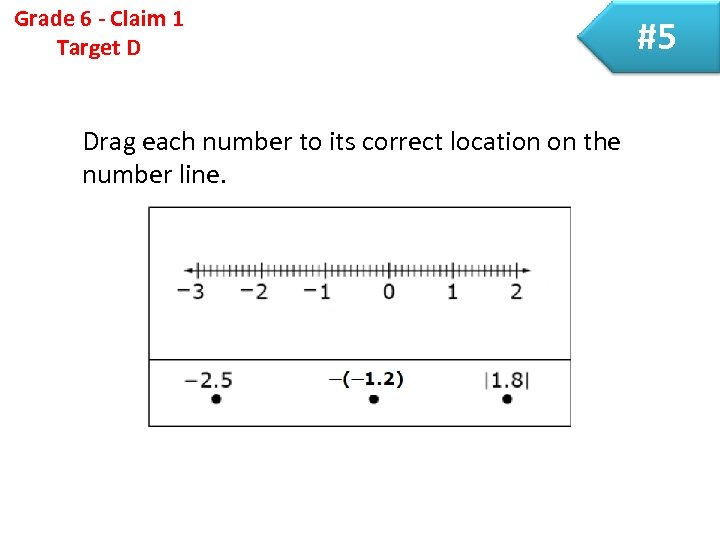Grade 6 - Claim 1 Target D Drag each number to its correct location on the number line. #5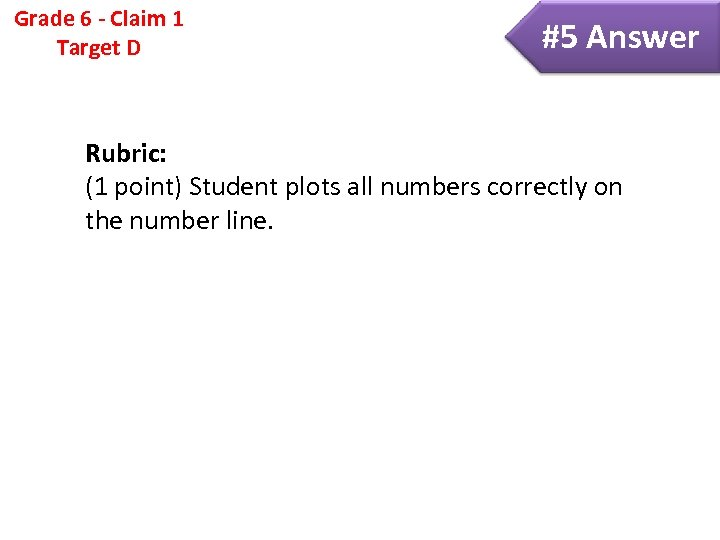Grade 6 - Claim 1 Target D #5 Answer Rubric: (1 point) Student plots all numbers correctly on the number line.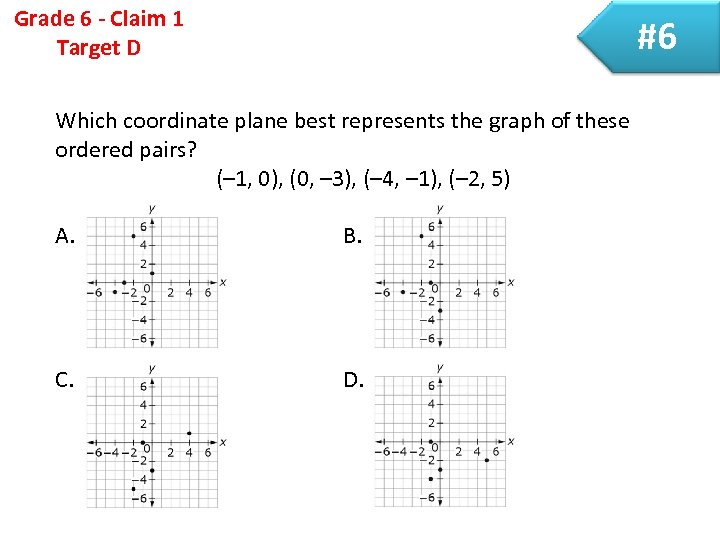Grade 6 - Claim 1 Target D #6 Which coordinate plane best represents the graph of these ordered pairs? (– 1, 0), (0, – 3), (– 4, – 1), (– 2, 5) A. B. C. D.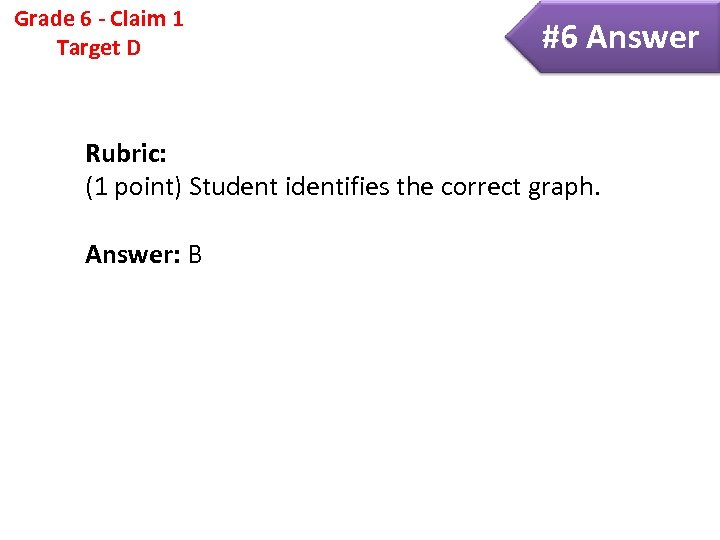Grade 6 - Claim 1 Target D #6 Answer Rubric: (1 point) Student identifies the correct graph. Answer: B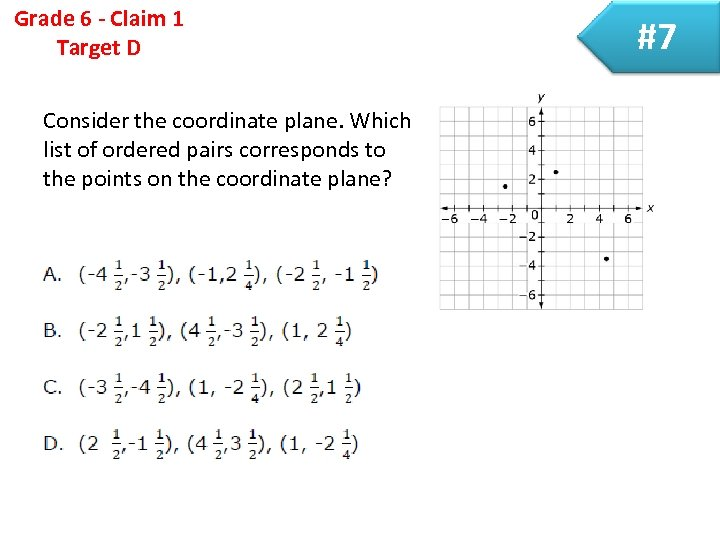Grade 6 - Claim 1 Target D Consider the coordinate plane. Which list of ordered pairs corresponds to the points on the coordinate plane? #7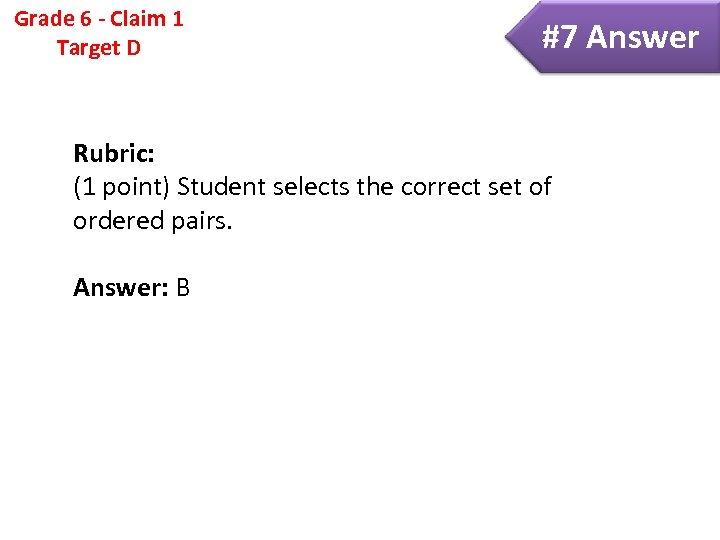Grade 6 - Claim 1 Target D #7 Answer Rubric: (1 point) Student selects the correct set of ordered pairs. Answer: B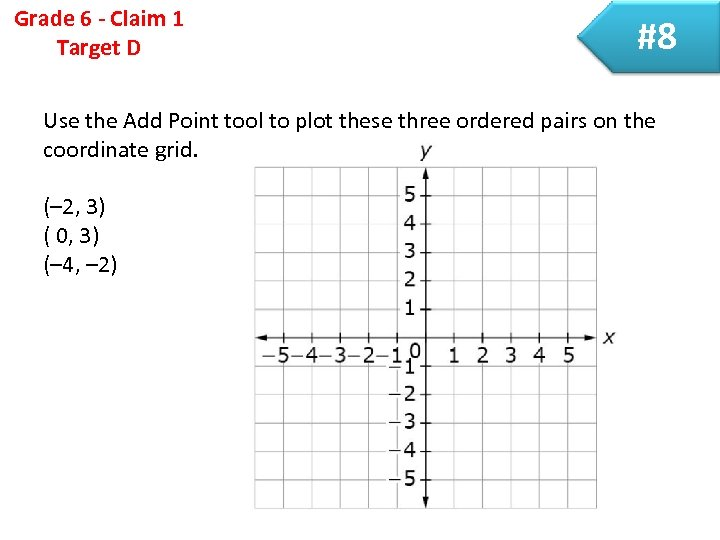Grade 6 - Claim 1 Target D #8 Use the Add Point tool to plot these three ordered pairs on the coordinate grid. (– 2, 3) ( 0, 3) (– 4, – 2)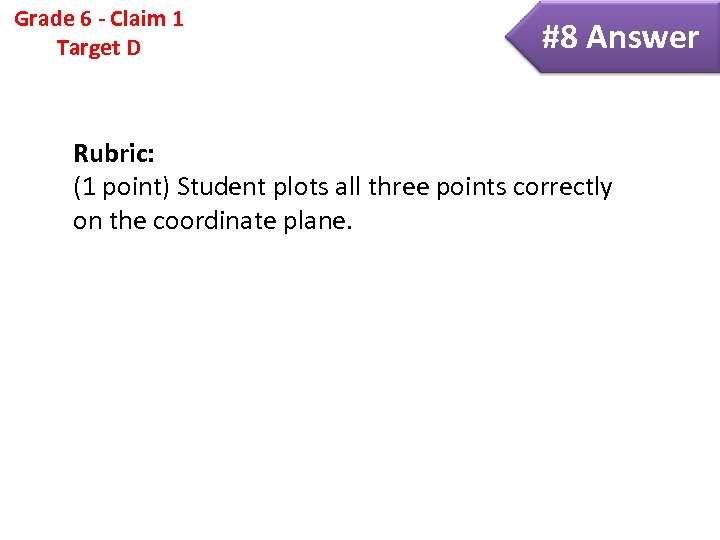Grade 6 - Claim 1 Target D #8 Answer Rubric: (1 point) Student plots all three points correctly on the coordinate plane.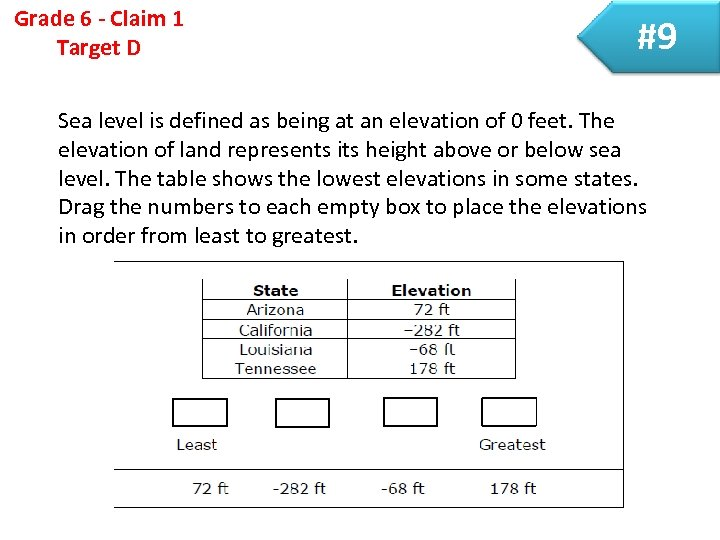Grade 6 - Claim 1 Target D #9 Sea level is defined as being at an elevation of 0 feet. The elevation of land represents its height above or below sea level. The table shows the lowest elevations in some states. Drag the numbers to each empty box to place the elevations in order from least to greatest.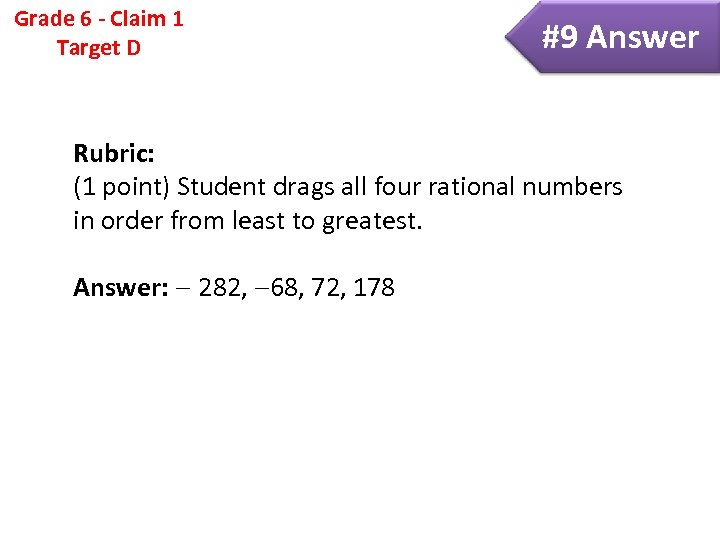Grade 6 - Claim 1 Target D #9 Answer Rubric: (1 point) Student drags all four rational numbers in order from least to greatest. Answer: 282, 68, 72, 178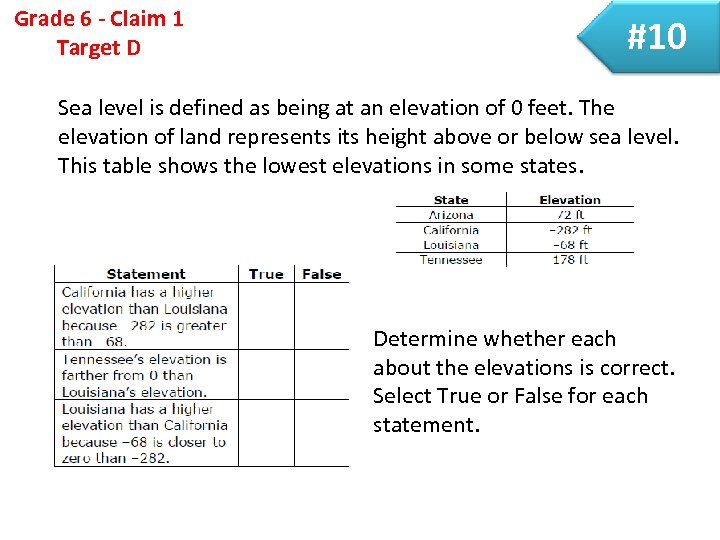Grade 6 - Claim 1 Target D #10 Sea level is defined as being at an elevation of 0 feet. The elevation of land represents its height above or below sea level. This table shows the lowest elevations in some states. statement Determine whether each about the elevations is correct. Select True or False for each statement.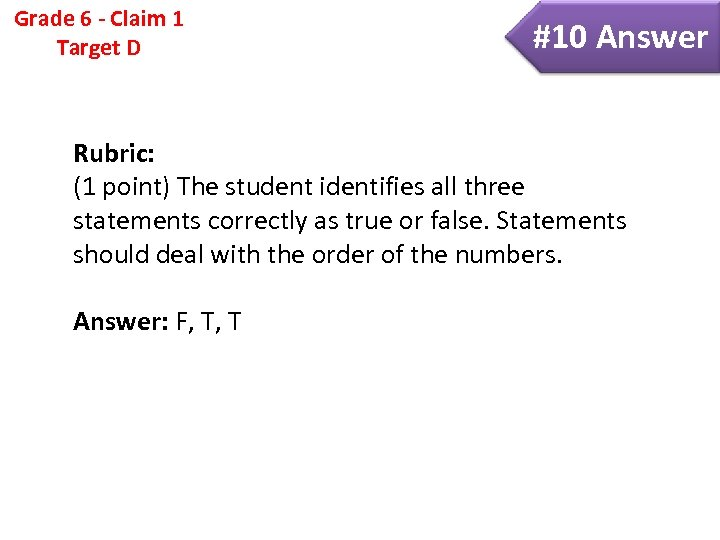Grade 6 - Claim 1 Target D #10 Answer Rubric: (1 point) The student identifies all three statements correctly as true or false. Statements should deal with the order of the numbers. Answer: F, T, T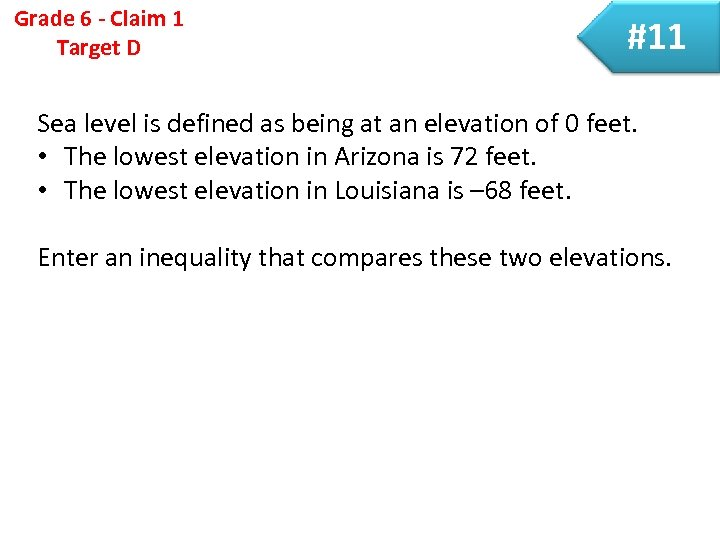Grade 6 - Claim 1 Target D #11 Sea level is defined as being at an elevation of 0 feet. • The lowest elevation in Arizona is 72 feet. • The lowest elevation in Louisiana is – 68 feet. Enter an inequality that compares these two elevations.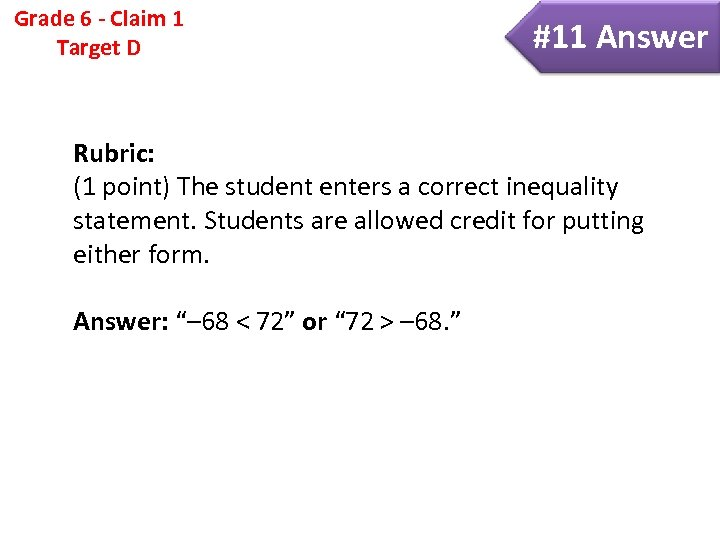Grade 6 - Claim 1 Target D #11 Answer Rubric: (1 point) The student enters a correct inequality statement. Students are allowed credit for putting either form. Answer: “– 68 < 72” or “ 72 > – 68. ”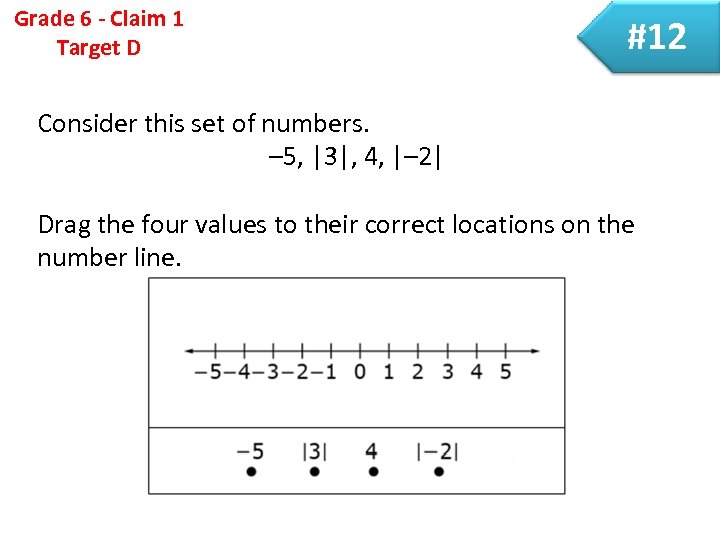Grade 6 - Claim 1 Target D #12 Consider this set of numbers. – 5, |3|, 4, |– 2| Drag the four values to their correct locations on the number line.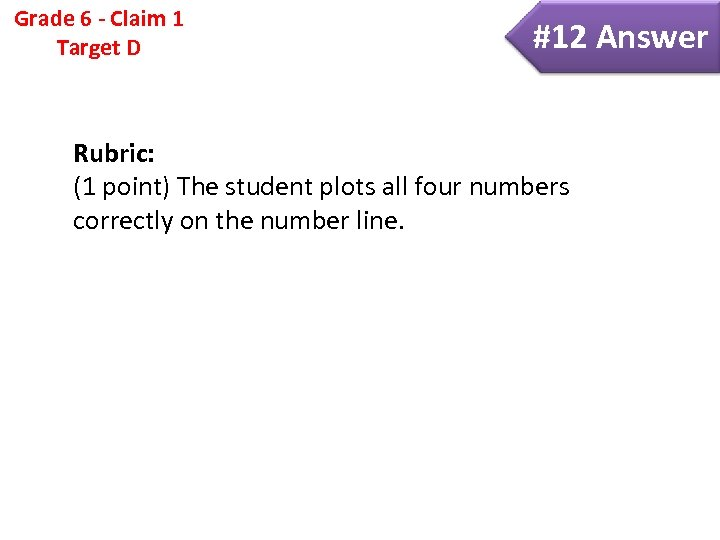Grade 6 - Claim 1 Target D #12 Answer Rubric: (1 point) The student plots all four numbers correctly on the number line.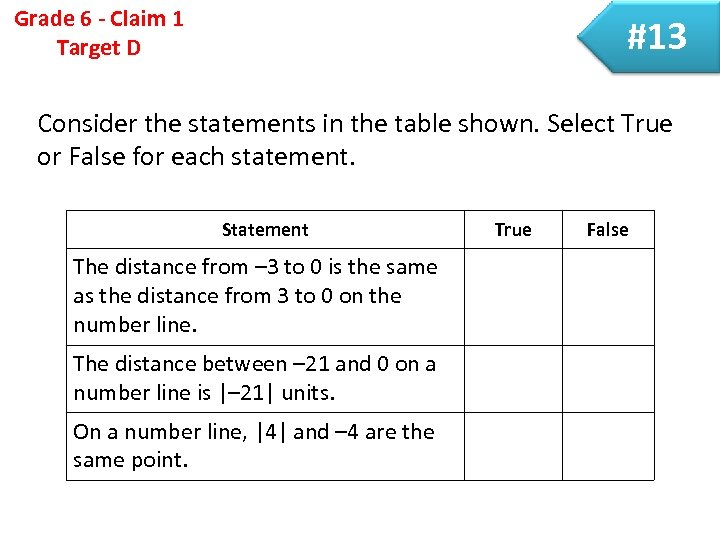Grade 6 - Claim 1 Target D #13 Consider the statements in the table shown. Select True or False for each statement. Statement The distance from – 3 to 0 is the same as the distance from 3 to 0 on the number line. The distance between – 21 and 0 on a number line is |– 21| units. On a number line, |4| and – 4 are the same point. True False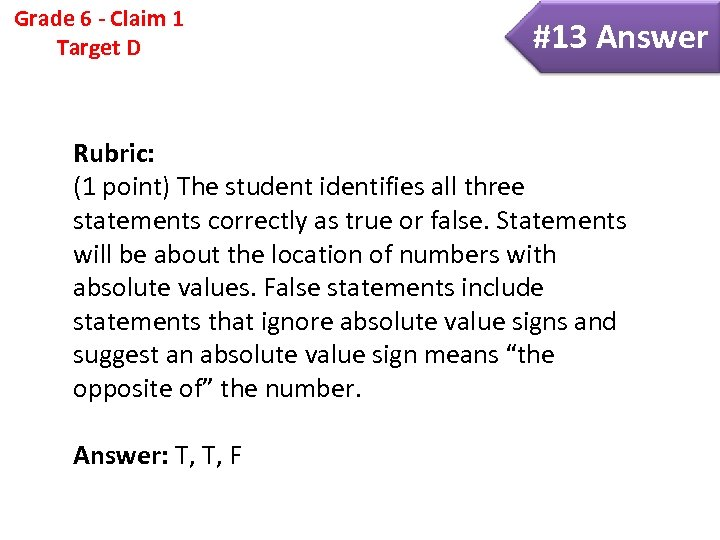Grade 6 - Claim 1 Target D #13 Answer Rubric: (1 point) The student identifies all three statements correctly as true or false. Statements will be about the location of numbers with absolute values. False statements include statements that ignore absolute value signs and suggest an absolute value sign means “the opposite of” the number. Answer: T, T, F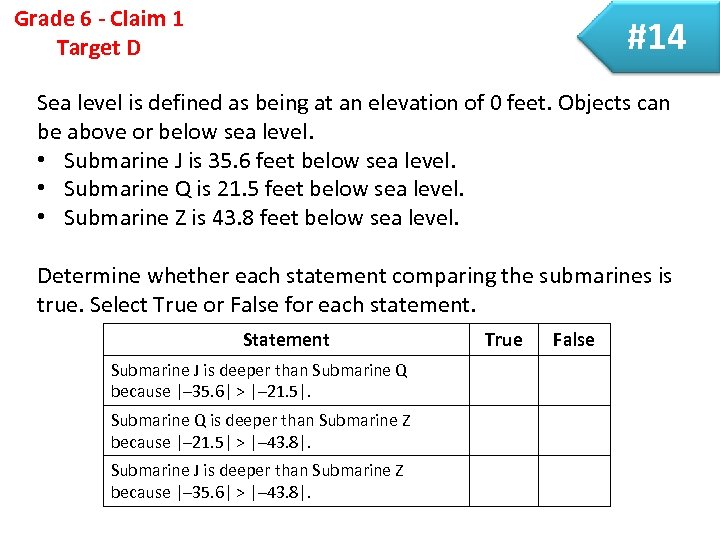Grade 6 - Claim 1 Target D #14 Sea level is defined as being at an elevation of 0 feet. Objects can be above or below sea level. • Submarine J is 35. 6 feet below sea level. • Submarine Q is 21. 5 feet below sea level. • Submarine Z is 43. 8 feet below sea level. Determine whether each statement comparing the submarines is true. Select True or False for each statement. Statement Submarine J is deeper than Submarine Q because |– 35. 6| > |– 21. 5|. Submarine Q is deeper than Submarine Z because |– 21. 5| > |– 43. 8|. Submarine J is deeper than Submarine Z because |– 35. 6| > |– 43. 8|. True False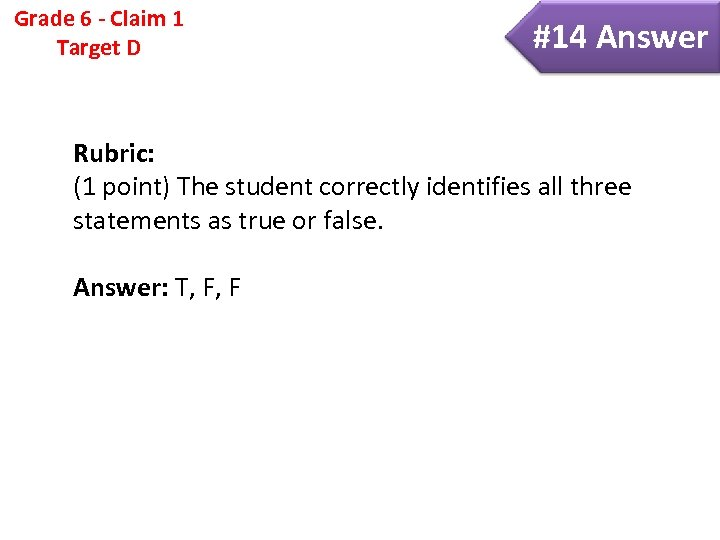Grade 6 - Claim 1 Target D #14 Answer Rubric: (1 point) The student correctly identifies all three statements as true or false. Answer: T, F, F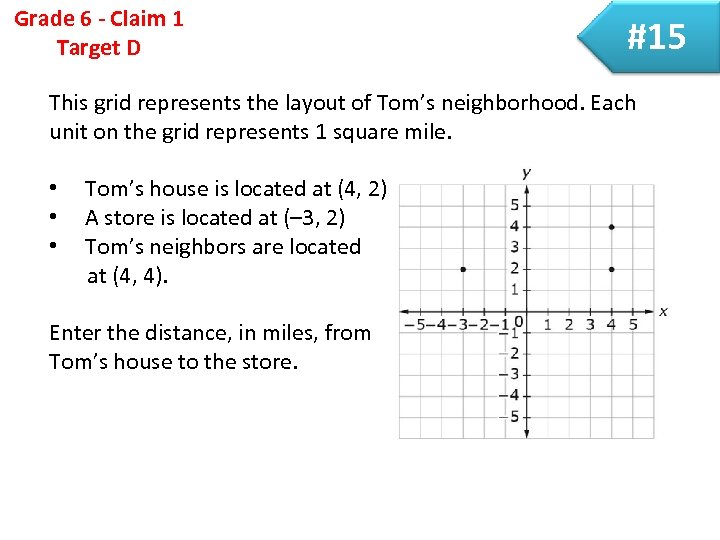Grade 6 - Claim 1 Target D #15 This grid represents the layout of Tom’s neighborhood. Each unit on the grid represents 1 square mile. • • • Tom’s house is located at (4, 2) A store is located at (– 3, 2) Tom’s neighbors are located at (4, 4). Enter the distance, in miles, from Tom’s house to the store.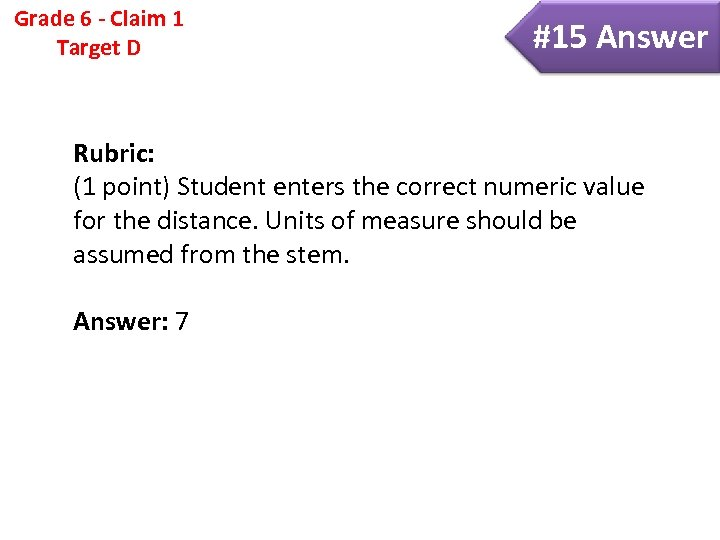Grade 6 - Claim 1 Target D #15 Answer Rubric: (1 point) Student enters the correct numeric value for the distance. Units of measure should be assumed from the stem. Answer: 7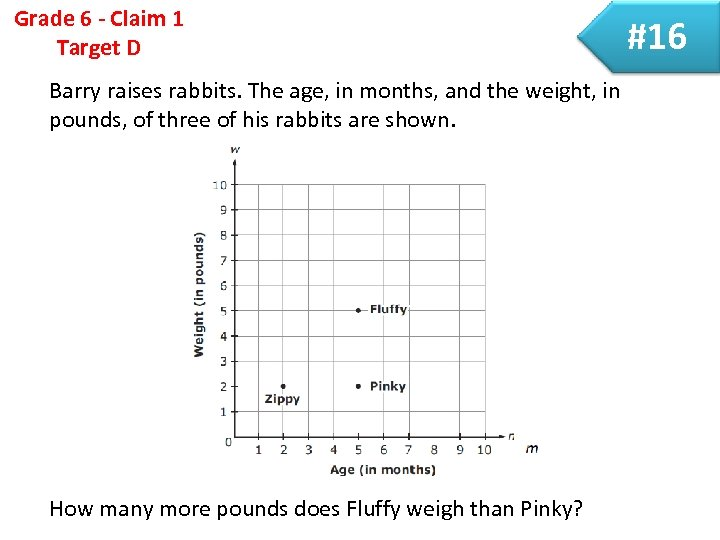Grade 6 - Claim 1 Target D Barry raises rabbits. The age, in months, and the weight, in pounds, of three of his rabbits are shown. How many more pounds does Fluffy weigh than Pinky? #16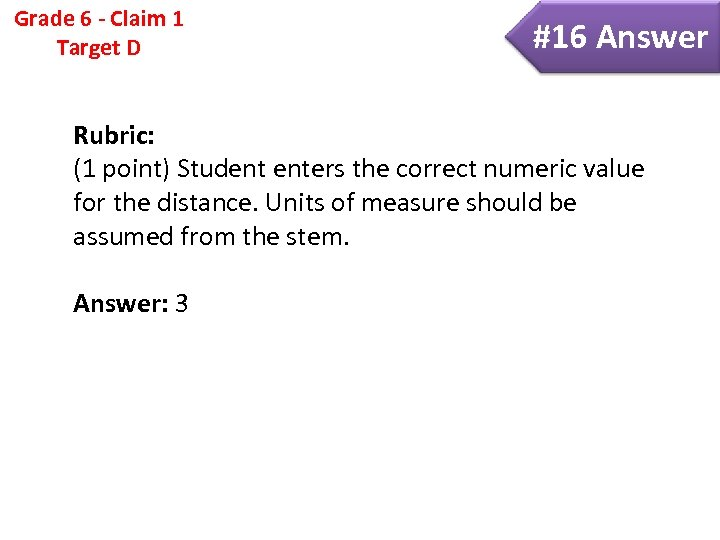Grade 6 - Claim 1 Target D #16 Answer Rubric: (1 point) Student enters the correct numeric value for the distance. Units of measure should be assumed from the stem. Answer: 3